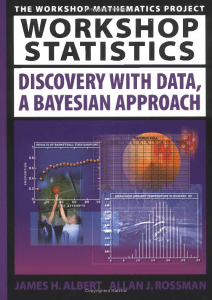21
Jan 17

## Review of Albert and Rossman

###Who is this book for?

In this review I concentrate on how this book is similar to and different from  Agresti and Franklin. The book contains almost no formulas and in this respect is even more basic than Agresti and Franklin. The emphasis of the book is on the Bayesian approach, which is not mainstream Statistics, and this makes it stand out from the crowd. The advantages of this emphasis are described on p.11 (avoiding the notion of a sampling distribution, making the course shorter and doing with less recipes).

### What I like

The text is business-like. Just one page (p.1) explains the difference between descriptive and inferential statistics, without even mentioning these names.

The book urges the instructor to reduce the amount of lecturing and rely more on active learning. It often prompts the student to think about ideas before providing the theoretical answer. That's what I like to do in my class. Activity 3-12 (Wrong Conclusions) pursues the same purpose.

The description of basic features of a data distribution on p.22 is concise and clear.

### What I don't like

The definition of a categorical variable (p.5) does not allow one to distinguish it from a numerical one. See my explanation.

No attempt is made to improve students' algebra skills.  This is what undermines the attempt to explain the Bayesian approach.

Like Agresti and Franklin, the authors make the study of regression dependent on the correlation coefficient. See Correlation and regression are two separate entities.

The logical sequence is broken. In particular, probabilities are introduced after regression.

The normal distribution, one of the pillars of Statistics, is given too late (in Chapter 18).

### Conclusion

As much as I like the idea of active learning, I cannot recommend the book, for the simple reason that it doesn't comply with the College Board curriculum.

Not being a Bayesian specialist, I was hoping to pick up something useful for myself. That hope didn't realize. The five chapters on the Bayesian approach are nothing more than just a collection of recipes accompanied by numerical examples. Even the Bayes theorem is not stated. If I were to write such a book, I would write it as a complement to a widely adopted text. This would allow me to avoid repeating the common stuff (graphical illustration of statistical data, measures of center and spread, probabilities etc.) and give more theory.

26
Nov 16

## Properties of correlation

### Correlation coefficient: the last block of statistical foundation

Correlation has already been mentioned in

Statistical measures and their geometric roots

Properties of standard deviation

The pearls of AP Statistics 35

Properties of covariance

The pearls of AP Statistics 33

### The hierarchy of definitions

Suppose random variables$X,Y$ are not constant. Then their standard deviations are not zero and we can define their correlation as in Chart 1.Chart 1. Correlation definition

### Properties of correlation

Property 1. Range of the correlation coefficient: for any$X,Y$ one has$- 1 \le \rho (X,Y) \le 1$.
This follows from the Cauchy-Schwarz inequality, as explained here.

Recall from this post that correlation is cosine of the angle between$X-EX$ and$Y-EY$.
Property 2. Interpretation of extreme cases. (Part 1) If$\rho (X,Y) = 1$, then$Y = aX + b$ with$a > 0.$

(Part 2) If$\rho (X,Y) = - 1$, then$Y = aX + b$ with$a < 0$.

Proof. (Part 1)$\rho (X,Y) = 1$ implies
(1)$Cov (X,Y) = \sigma (X)\sigma (Y)$
which, in turn, implies that$Y$ is a linear function of$X$:$Y = aX + b$ (this is the second part of the Cauchy-Schwarz inequality). Further, we can establish the sign of the number$a$. By the properties of variance and covariance$Cov(X,Y)=Cov(X,aX+b)=aCov(X,X)+Cov(X,b)=aVar(X)$,$\sigma (Y)=\sigma(aX + b)=\sigma (aX)=|a|\sigma (X)$.
Plugging this in Eq. (1) we get$aVar(X) = |a|\sigma^2(X)$ and see that$a$ is positive.

The proof of Part 2 is left as an exercise.

Property 3. Suppose we want to measure correlation between weight$W$ and height$H$ of people. The measurements are either in kilos and centimeters${W_k},{H_c}$ or in pounds and feet${W_p},{H_f}$. The correlation coefficient is unit-free in the sense that it does not depend on the units used:$\rho (W_k,H_c)=\rho (W_p,H_f)$. Mathematically speaking, correlation is homogeneous of degree$0$ in both arguments.
Proof. One measurement is proportional to another,$W_k=aW_p,\ H_c=bH_f$ with some positive constants$a,b$. By homogeneity$\rho (W_k,H_c)=\frac{Cov(W_k,H_c)}{\sigma(W_k)\sigma(H_c)}=\frac{Cov(aW_p,bH_f)}{\sigma(aW_p)\sigma(bH_f)}=\frac{abCov(W_p,H_f)}{ab\sigma(W_p)\sigma (H_f)}=\rho (W_p,H_f).$